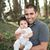# Alok Menghrajani

Previously: security engineer at Square, co-author of HackLang, put the 's' in https at Facebook. Maker of CTFs.

This blog does not use any tracking cookies and does not serve any ads. Enjoy your anonymity; I have no idea who you are, where you came from, and where you are headed to. Let's dream of an Internet from times past.

Most programming languages offer various bit level manipulation operators (bitwise and, bitwise or, shifting to the right or to the left, etc.).

Puzzles like this one are a great way to learn about the bit level representation of numbers and the various bit related operators.

This puzzle requires you to implement a piece of code with only a limited set of operators.

Your solution to the puzzles gets validated automatically and your score is based on the total length of your code (lower score is better).

Allowed operators: `˜ & ^ | + << >> >>>`

Write a function in JavaScript which returns 1 if x contains exactly one bit set to 1. It should return 0 if x is zero or contains more than one bit set to 1.

Your solution can only use assignments and the above list of operators. You cannot use any conditional (`if`) statements, or loops (no `for`, `while`, etc.).

You can assign intermediate results to as many variables as you need (assignments don't count towards your score) and you can use constants in the 0-255 range.

Go ahead, and write your code below. Hit validate when you are done!

function one_bit(x) { // write your code here // end it with a return statement }

Aug 31, 2016: Tom Theisen for sending me a better solution.

Jun 29, 2019: Royce Rajan crushed the previous best solution!

Here is some information on what each operator does:

 `˜x` (bitwise not): Flips all the bits in x. `x&y` (bitwise and): For each bit in x,y, sets the result's bit to 1 if both x and y have their bit set. `x^y` (bitwise xor): For each bit in x,y, sets the result's bit to 1 if x or y, but not both, have their bit set. `x|y` (bitwise or): For each bit in x,y, sets the result's bit to 1 if x or y have their bit set. `x+y` (addition): Adds x and y. `x<>n` (bitwise shift right): Shifts the bits in x to the right, by n bits. The sign is maintained. `x>>>n` (logical shift right): Shifts the bits in x to the right, by n bits. Zeros are filled in from the left.

Note: A useful trick is `-x = ~x+1`!

Fun fact: JavaScript is a funny beast, the language does not really expose integers (all math operators return numerical values). The language does implement these various bit manipulation operators, which treat their operands as 32 bits (and return a numerical value).

More similar puzzles:

And a rich collection of hacks:

Sometimes you use this kind of trick in production code.